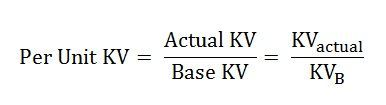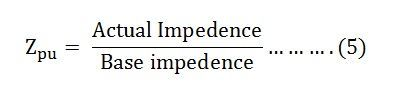# Per Unit System

For the analysis of electrical machines or electrical machine system, different values are required, thus, per unit system provides the value for voltage, current, power, impendence, and admittance. The Per Unit System also makes the calculation easier as all the values are taken in the same unit. The per-unit system is mainly used in the circuit where variation in voltage occurs.

Contents:

Definition: The per-unit value of any quantity is defined as the ratio of actual value in any unit to the base or reference value in the same unit. Any quantity is converted into per unit quantity by dividing the numeral value by the chosen base value of the same dimension. The per-unit value is dimensionless.The base values can be selected arbitrarily. It is usual to assume the base values as given below

• Base voltage = rated voltage of the machine
• Base current = rated current of the machine
• Base impedance = base voltage /base current
• Base power = base voltage x base current

Firstly the value of base power and the base voltage are selected, and their choice automatically fixes the other base values.
AsSo,Putting the value of base current from the equation (1) in equation (2) we getPutting the value of base current from the equation (1) in the equation (3) we getNow,Putting the value of base impedance from the equation (4) in the equation (5) we will get the value of impedance per unit

## Advantages of Per Unit System

There are mainly two advantages of using the Per Unit System.

• The parameters of the rotating electrical machines and the transformer lie roughly in the same range of numerical values, irrespective of their ratings if expressed in a per-unit system of ratings.
• It relieves the analyst of the need to refer circuit quantities to one or the other side of the transformer, making the calculations easy.

Taking the example of a transformer having the resistance in the per unit as Rpu ohm and the reactance as Xpu in ohm with referred to primary then per unit values will beWhere Rep and Xep are resistance and reactance referred to primary and pu means in the per-unit system.

The resistance and leakage reactance referred to primary in per unit are the same as referred to as secondary in the per-unit system becauseWhere Res and Xes are the equivalent resistance and reactance referred to secondary

Therefore, from the above two equations, it is clear that the need for an ideal transformer is eliminated. This is so because the per-unit impedance is the same for the equivalent circuit of the transformer whether computed from the primary or secondary as long as the voltage bases on the two sides are selected in the ratio of transformation.

### 9 thoughts on “Per Unit System”

1. its a very useful explanation about per unit system.
Thanks a lot for your efforts.
Best regards: Reza tousipanah